# IB DP Physics: SL复习笔记3.1.7 Investigating Thermal Energy

### Investigating Thermal Energy

#### Aim of the Experiment

• The aim of the experiment is to determine the specific heat capacity of a metal block

Variables

• Independent variable = Time, t (s)
• Dependent variable = Temperature, T (°C)
• Control variables:
• Mass of the metal block
• Voltage of the power supply

####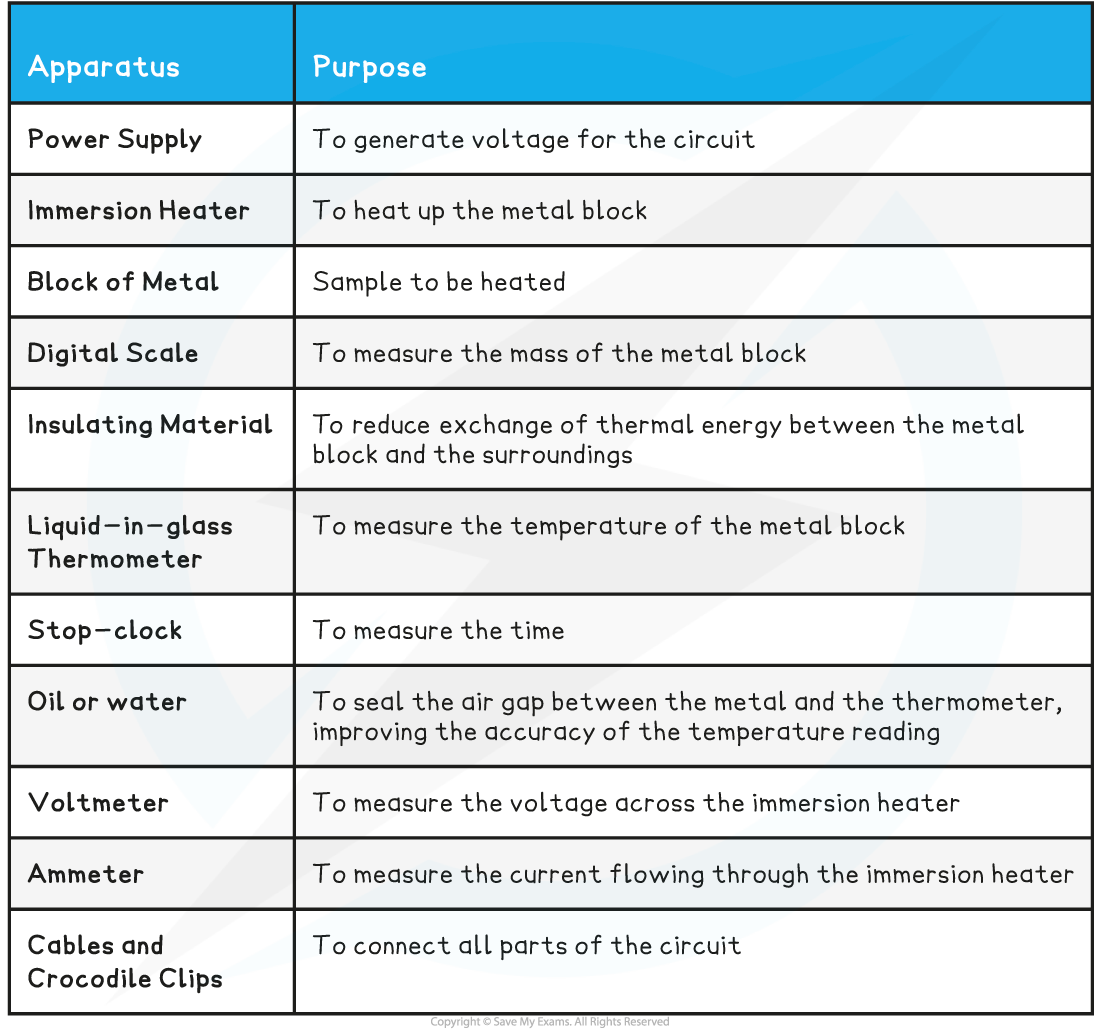• Resolution of measuring equipment:
• Digital scale = 0.1 g
• Liquid-in-glass thermometer = 1°C
• Stop-clock = 0.01 s
• Voltmeter = 1 mV
• Ammeter = 1 mA

#### Method

Part 1: Measuring the temperature change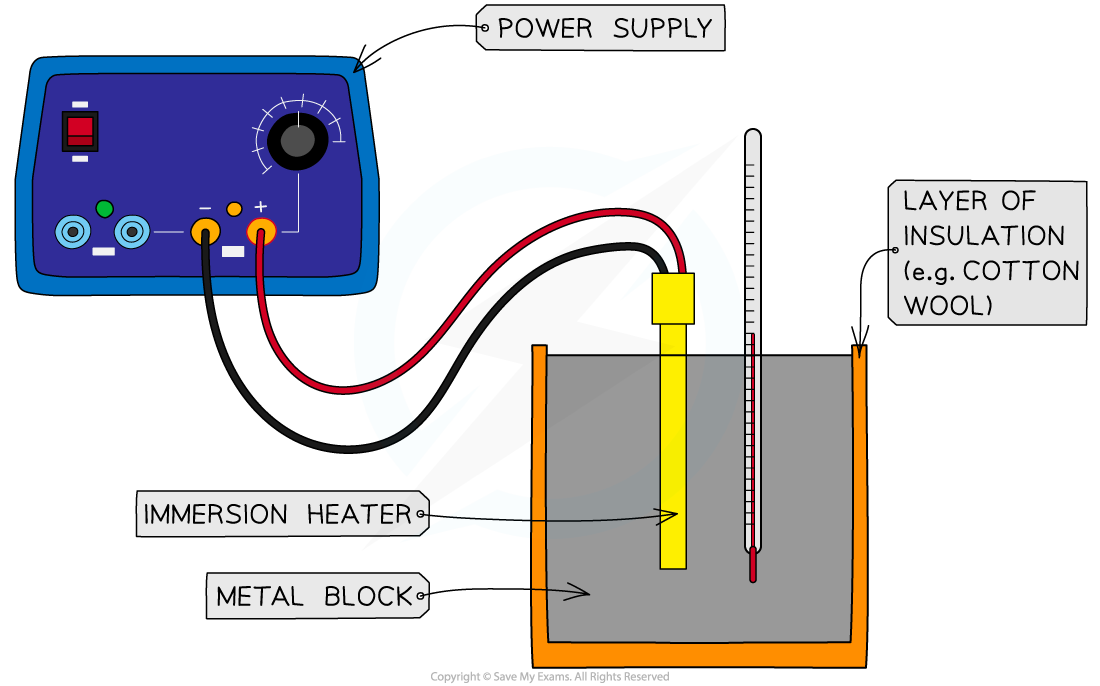Apparatus used to investigate the specific heat capacity of a metal block

1. Use the digital scale to measure the mass m of the metal block. Record this value with its uncertainty Δm (this is simply the smallest division on the digital scale - e.g. ± 0.0001 kg)
2. Wrap the insulating material (e.g. cotton wool) around the block of metal to minimise energy transfers with the surroundings
3. Insert the immersion heater into the central hole of the metal block
4. Place the thermometer into the smaller hole in the metal block
5. Put a few drops of oil or water into the hole where the thermometer is, to seal the air gap between the metal and the thermometer
6. Measure the temperature T of the metal block and record this value in the first row of the table (t = 0), together with its uncertainty ΔT
7. Connect the immersion heater to the power supply
8. Turn on the power supply and start the stop-clock at the same time
9. Measure the temperature of the block every minute (i.e. 60 seconds) and record the temperature values in the table (for a total of 10 readings)
10. Turn the immersion heater off
• An example of a results table might look like this: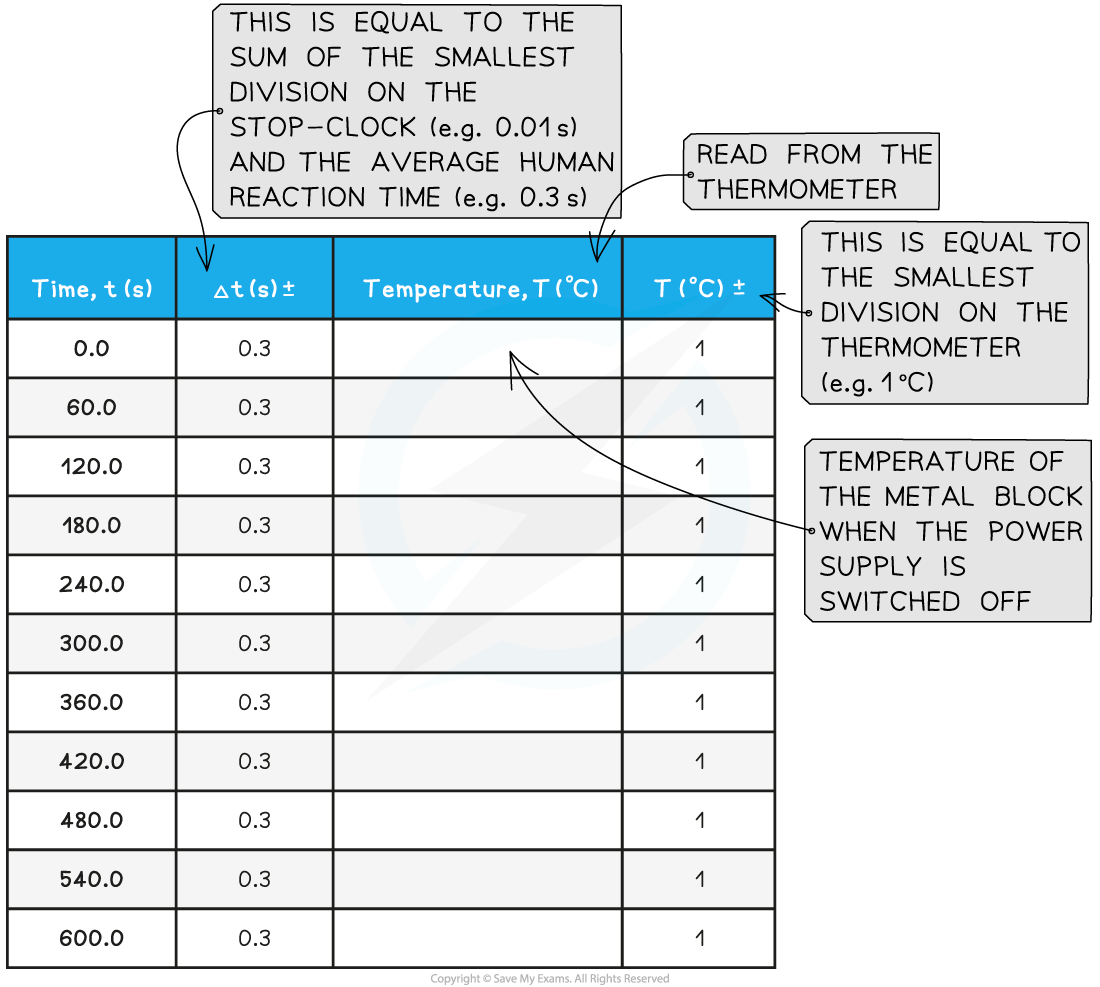Part 2: Calculating the energy transferred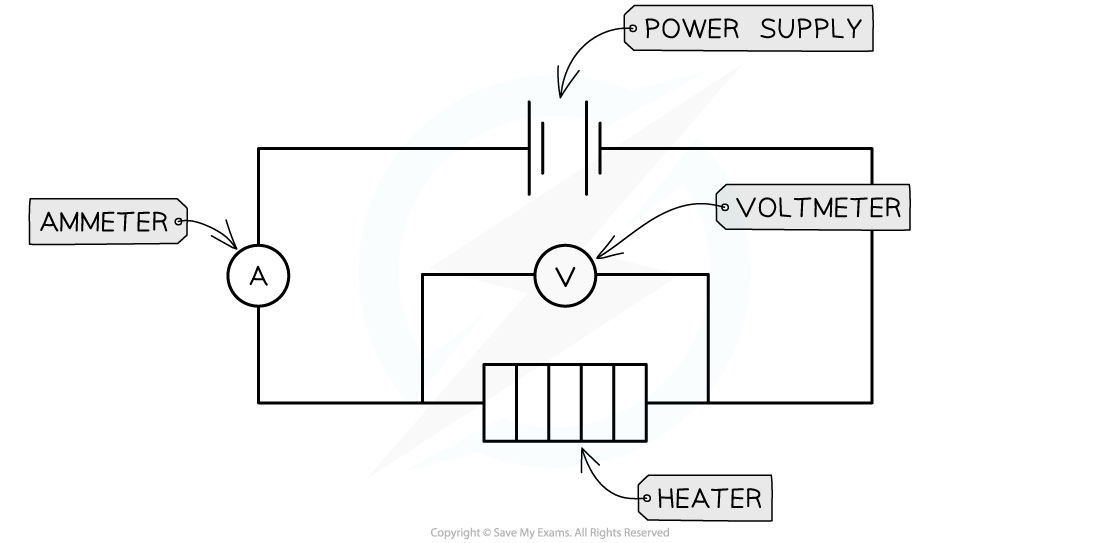Apparatus used to measure the energy transferred to the metal block

1. Connect the ammeter in series with the immersion heater and the voltmeter across the immersion heater (i.e. in parallel)
2. Turn on the power supply using the same value of voltage used to take the readings of temperature
3. Read the value of the current I from the ammeter and record this value with its uncertainty ΔI
4. Read the value of the voltage V from the voltmeter (not from the power supply) and record this value with its uncertainty ΔV
• An example of a results table might look like this:#### Analysis of Results

• The equation linking the thermal energy E transferred to the metal block, the mass m of the block, the specific heat capacity c of the block and the temperature change ΔT is:

E = mcΔT

• Rearrange this to calculate the specific heat capacity c: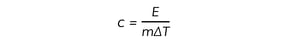• Write the energy E in terms of power P and time Δt:

E = PΔt

• Substitute this expression for E into the equation for the specific heat capacity:• Write the power P as the product of voltage V and current I: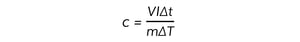• Plot a graph of temperature T (°C) against time t (s)
• Calculate the gradient of the linear portion of the graph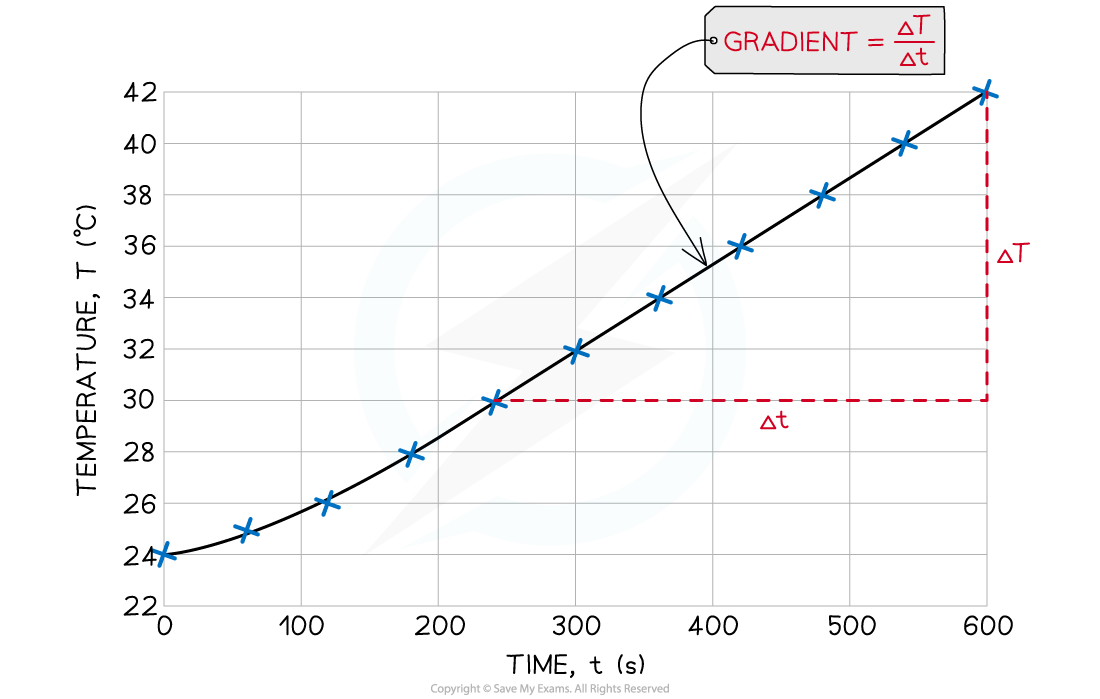• Calculate the specific heat capacity c of the metal (in J kg–1 K–1 or, equivalently, in J kg–1 °C–1) as follows: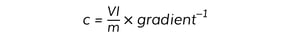• Compare this result with the accepted value of the specific heat capacity for the metal used

#### Evaluating the Experiment

Systematic errors:

• Some energy is lost to the surroundings
• The layer of insulation around the metal block and the drops of oil or water in the hole where the thermometer is placed all help reducing energy losses, but some energy is inevitably transferred to the surroundings, causing a systematic error
• The thermometer might not be calibrated correctly
• Place the thermometer in a beaker with a mixture of ice and water for 30 s, of the thermometer reads 0°C, then it is reading correctly and can be used

Random errors:

• There might be parallax error when reading the values of temperature from the thermometer
• Make sure you hold the thermometer at eye level when taking the temperature readings to reduce random error
• The temperature readings might not all be taken exactly 60 s apart from each other
• Make sure you work with a partner, so you can read out the temperature values to them and they can write or type these in the table, while you are only looking at the stop-clock and thermometer
• Delays in the responsiveness of the thermometer might still cause random errors, using a thermometer with a smaller bulb and thinner glass walls will improve its responsiveness

#### Safety Considerations

• Position all equipment away from the edge of the desk
• Do not touch the metal block or the immersion heater when the power supply is switched on
• Allow time to cool before touching hot parts of the apparatus

#### Worked Example

Use error analysis to obtain expressions for the relative error δc and the absolute error Δc on the specific heat capacity.

Step 1: Determine the relative errors on voltage V, current I, temperature T, mass m and time t

• δV ΔV/V
• δI ΔI/I
• δT ΔT/(where T is the increase in temperature for the straight portion of the graph)
• δm = Δm/m
• δt = Δt/(where t is the increase in time for the straight portion of the graph)

Step 2: Sum all relative errors in Step 1 to obtain the relative error δc on the specific heat capacity

δc = δV + δI + δT + δm + δt

Step 3: Recall the relationship between absolute and relative errors to determine the absolute error on the specific heat capacity Δc

• Remember that absolute errors have units (in this case, J kg–1 K–1 or in J kg–1 °C–1)
• c in the equation below is the experimental value of the specific heat capacity

Δc = c × δc